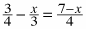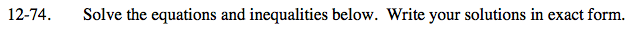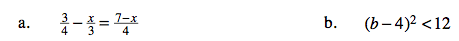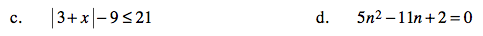### Home > CA > Chapter Ch12 > Lesson 12.4.2 > Problem12-74

12-74.
1. Solve the equations and inequalities below. Write your solutions in exact form. Homework Help ✎

1.2. (b − 4)2 < 12

3. | 3 + x | − 9 < 21

4. 5n2 − 11n + 2 = 0Multiply the equation by a common multiple of the denominators to get rid of the fraction.

$12\left(\frac{3}{4}-\frac{x}{3}=\frac{7-x}{4}\right)$

9 − 4x = 21 − 3x

Solve for x.

−12 = x

Find the boundary points.

Take the square root of both sides.

Solve for b.

Test numbers on all sides of the boundary point or points.

Write an inequality to express your answer.−33 ≤ x ≤ 27

Factor the equation.

Solve for n.